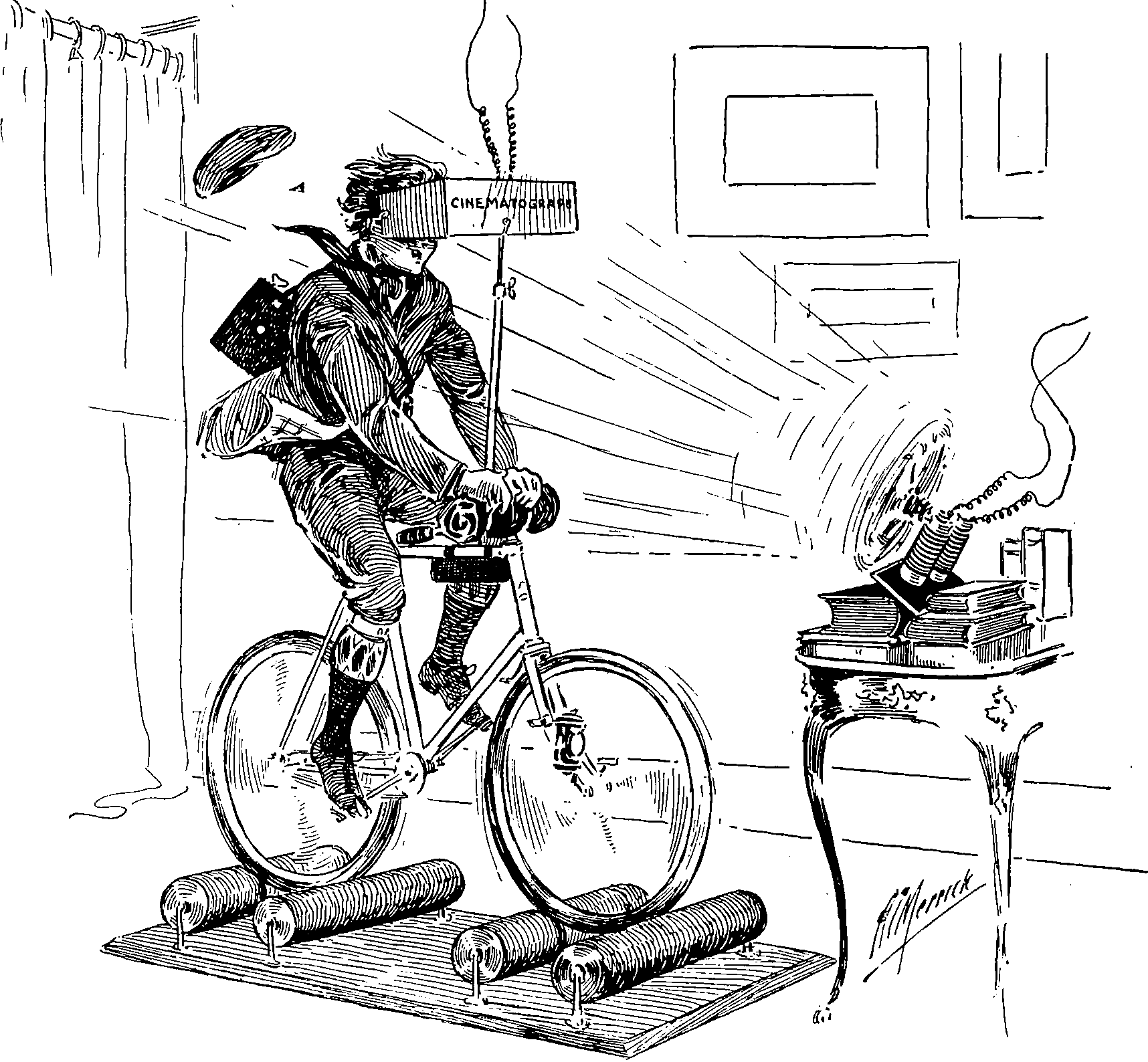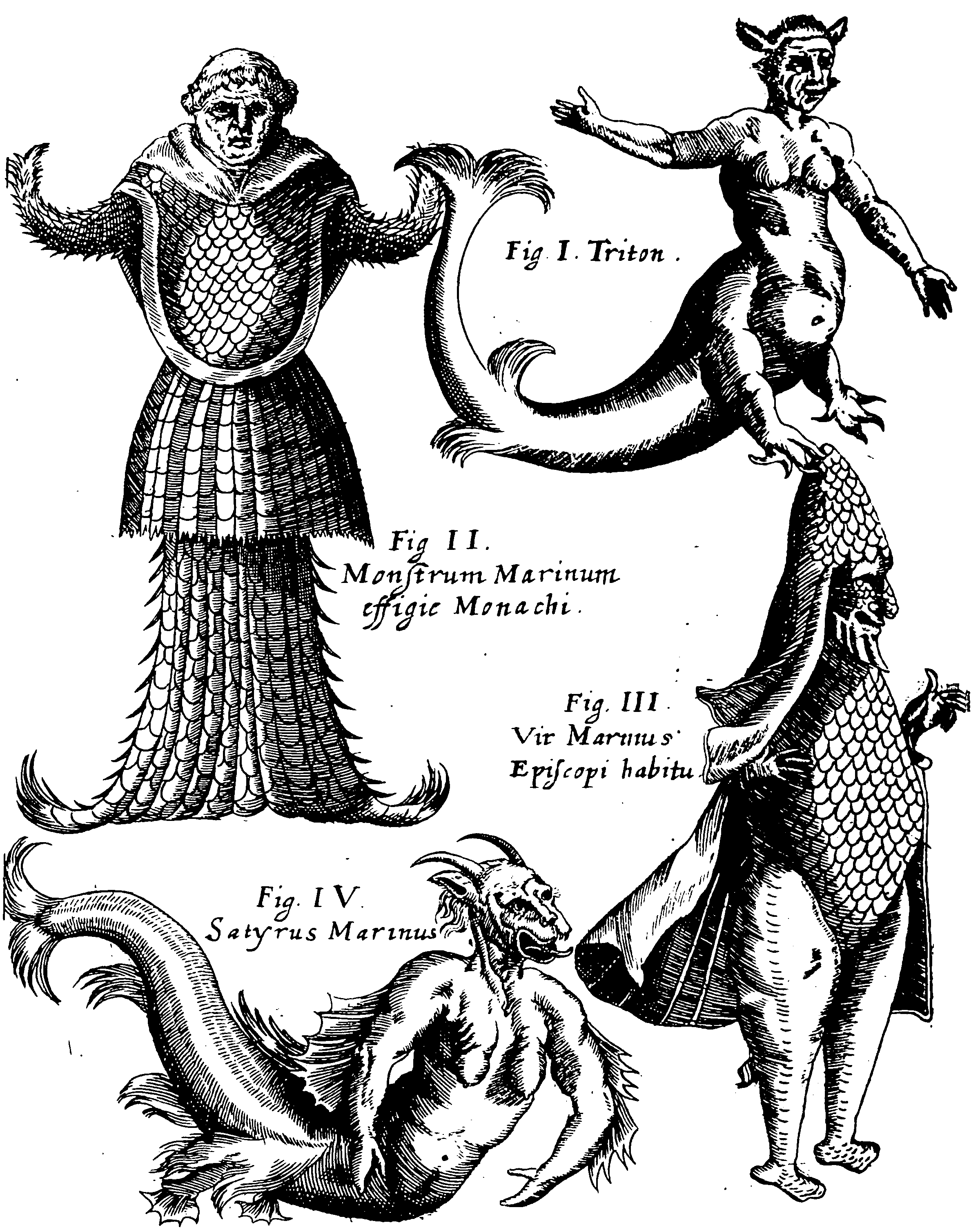# Warping of stationary stochastic processesTransforming stationary processes into non-stationary ones by transforming their inputs .

This is of interest in the context of composing kernels to have known desirable properties by known transforms, and also learning (somewhat) arbitrary transforms to attain stationarity.

One might consider instead processes that are stationary upon a manifold.

## Stationary reducible kernelsThe main idea is to find a new feature space where stationarity or local stationarity can be achieved.

Genton (2001) summarises:

We say that a nonstationary kernel $$K(\mathbf{x}, \mathbf{z})$$ is stationary reducible if there exist a bijective deformation $$\Phi$$ such that: $K(\mathbf{x}, \mathbf{z})=K_{S}^{*}(\mathbf{\Phi}(\mathbf{x})-\mathbf{\Phi}(\mathbf{z}))$ where $$K_{S}^{*}$$ is a stationary kernel.

## Classic deformations

### As a function of input

Invented apparently by Gibbs (1998) and generalised in Paciorek and Schervish (2003).

Let $$k_S$$ be some stationary kernel on $$\mathbb{R}^D.$$ Let $$\Sigma(\mathbf{x})$$ be a $$D \times D$$ matrix-valued function which is positive definite for all $$\mathbf{x},$$ and let $$\Sigma_{i} \triangleq \Sigma\left(\mathbf{x}_{i}\right) .$$ ) Then define $Q_{i j}=\left(\mathbf{x}_{i}-\mathbf{x}_{j}\right)^{\top}\left(\left(\Sigma_{i}+\Sigma_{j}\right) / 2\right)^{-1}\left(\mathbf{x}_{i}-\mathbf{x}_{j}\right)$ Then $k_{\mathrm{NS}}\left(\mathbf{x}_{i}, \mathbf{x}_{j}\right)=2^{D / 2}\left|\Sigma_{i}\right|^{1 / 4}\left|\Sigma_{j}\right|^{1 / 4}\left|\Sigma_{i}+\Sigma_{j}\right|^{-1 / 2} k_{\mathrm{S}}\left(\sqrt{Q_{i j}}\right)$ is a valid non-stationary covariance function.

Homework question: Is this a product of convolutional gaussian processes.

## Learning transforms### No comments yet. Why not leave one?

GitHub-flavored Markdown & a sane subset of HTML is supported.